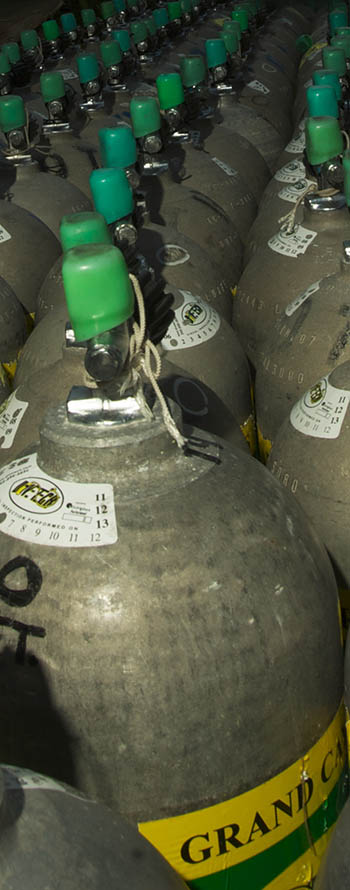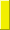DIRECTORY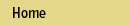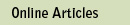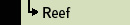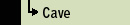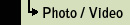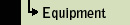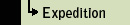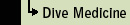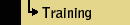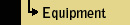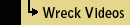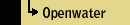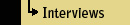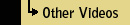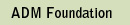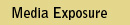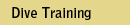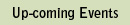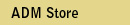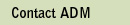By Curt Bowen Heliair, a term originally coined by Sheck Exley, is the simple process of adding helium to a scuba cylinder then topping it off with air. Heliair, sometimes called "poor man's mix" was implemented in the early deep air days of cave exploration for the reduction of nitrogen narcosis. At this time it was believed that narcosis was the cause of several deaths of divers using deep air. Today, we know that even though nitrogen narcosis may have been a contributing factor, most likely oxygen toxicity was the culprit. Heliair has multiple advantages and disadvantages for the extended range diver Advantages Nitrogen Narcosis: Heliair reduces the effects of nitrogen narcosis. Oxygen Toxicity: Heliair reduces the oxygen PO2 exposure levels reducing the possibility of a 02 hit. Ease of Mixing: Heliair mixture s are easily obtained by adding helium to a scuba cylinder then topping it off using a standard air compressor. No Fire Hazard: Heliair mixtures don 't require handling of 100% oxygen, thus removing any possible fire hazard. Analyzing: Heliair's nitrogen and helium percentage s are a mathematical constant according to the oxygen percentage thus providing the blender with the exact nitrogen and helium percentages. Ease of Travel: Heliair minimizes the need to carry additional bank cylinders of oxygen to remote dive locations. Continuous Blending: With the proper mixing chamber, heliair can be pumped directly through a standard compressor. Disadvantages: Best Mix: In trimix, a PO2 of l .4ata and an equivalent narcosis depth of 130 feet are desirable. With heliair, to obtain an equivalent narcosis depth of 130 feet, a PO2 of 0.9 to 1.1 ata must be accepted – far below the desired l.4ata PO2. If a PO2 of l .4 ata is obtained then an equivalent narcosis depth must be accepted, much deeper than the desired 130 feet. Increased Decompression: Due to the fact that optimal PO2s of 1.4 are not obtained, decompression requirements will increase slightly compared to that of the Best Mix. Partial Pressure Mixing: Two basic formulas are required to mix heliair. The first formula is used to determine how much helium in psi is needed for a desired mix. FHe x Ending Pressure, where FHe stands for fraction of helium. Example: An ending helium percentage of 24% is desired with an ending pressure of 2640 psi. Formula: 0.24 x 2640 = 633 psi helium to be added. The second formula gives the oxygen percentage in the cylinder after topping off with air: 1 - FHe x 0.21 Example: The oxygen percentage in the above 24% mix would be (1 - 0.24) x 0.21 = 0. 159 or 15.9% The mixing process is relatively simple. First drain the scuba cylinder then bank the required p i of helium into the scuba cylinder. Allow time for the helium to cool and the pressure 10 drop then make the needed corrections. Fill the scuba cylinder with air to the desired ending psi. Analyze the oxygen content with an 02 analyze r. If the oxygen percentage is too low just add more air until the mix is correct. Always round the oxygen percentage down (Example 15.6% to 15%) to the next lower number this will add a small amount of safety to the decompression tables.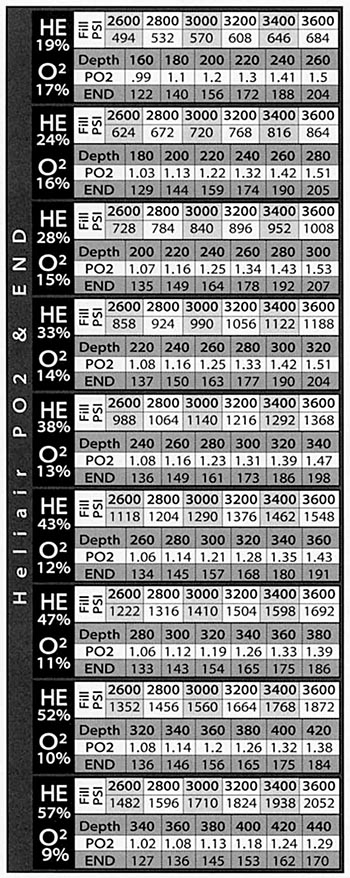This chart gives the Equivalent Narcosis Depth( END) in fsw, and the partial pressure of oxygen (P02) in atmospheres absolute for various heliair mixtures. Match up the desired depth with the desired END or P02. Once located, the desired helium and oxygen percentages are in the left black box. The helium fill psi from 2600 -3600 psi are located in the same block just above the depth row.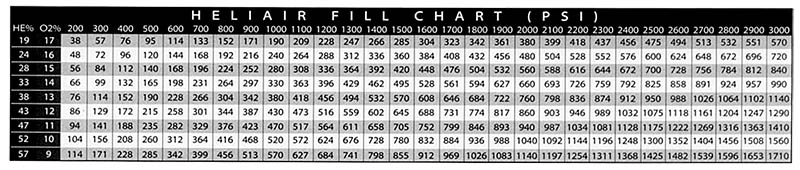This chart provides the amount of helium to add (in psi) to an empty scuba cylinder to create the various heliair mixtures. First, find the desired heliair mixture to the left, then the ending scuba cylinder pressure at the top, intersect these two rows to get the required helium (in psi). Then top off the tank with air. You can also use the chart to determine the amount of helium left in a cylinder after a dive. Many times this helium can be saved.